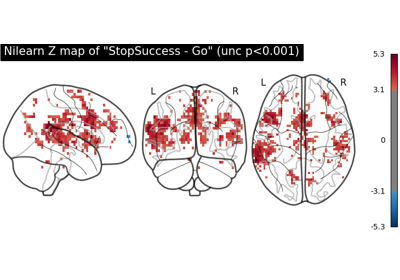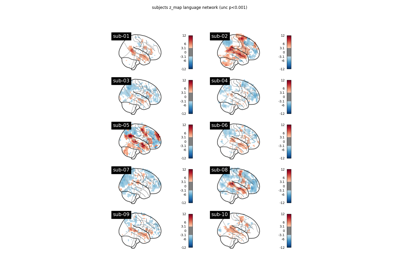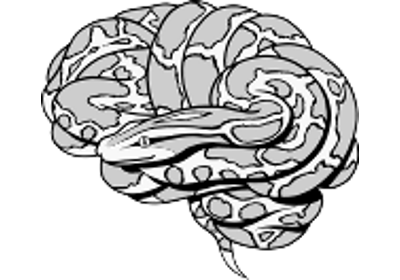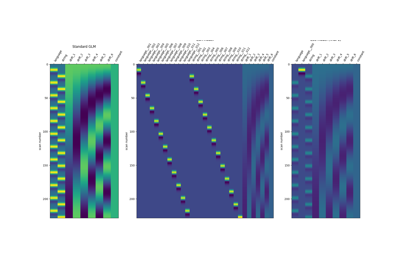Note

This page is a reference documentation. It only explains the function signature, and not how to use it. Please refer to the user guide for the big picture.

# nilearn.glm.first_level.first_level_from_bids#

nilearn.glm.first_level.first_level_from_bids(dataset_path, task_label, space_label=None, sub_labels=None, img_filters=None, t_r=None, slice_time_ref=0.0, hrf_model='glover', drift_model='cosine', high_pass=0.01, drift_order=1, fir_delays=, min_onset=-24, mask_img=None, target_affine=None, target_shape=None, smoothing_fwhm=None, memory=Memory(location=None), memory_level=1, standardize=False, signal_scaling=0, noise_model='ar1', verbose=0, n_jobs=1, minimize_memory=True, derivatives_folder='derivatives')[source]#

Create FirstLevelModel objects and fit arguments from a BIDS dataset.

If t_r is None this function will attempt to load it from a bold.json. If slice_time_ref is None this function will attempt to infer it from a bold.json. Otherwise t_r and slice_time_ref are taken as given, but a warning may be raised if they are not consistent with the bold.json.

Parameters:
dataset_path

Directory of the highest level folder of the BIDS dataset. Should contain subject folders and a derivatives folder.

task_label`str`

space_label`str`, optional

Specifies the space label of the preprocessed bold.nii images. As they are specified in the file names like _space-<space_label>_.

sub_labels`list` of `str`, optional

Specifies the subset of subject labels to model. If ‘None’, will model all subjects in the dataset. .. versionadded:: 0.10.1

img_filters`list` of `tuple` (str, str), optional

Filters are of the form (field, label). Only one filter per field allowed. A file that does not match a filter will be discarded. Possible filters are ‘acq’, ‘ce’, ‘dir’, ‘rec’, ‘run’, ‘echo’, ‘res’, ‘den’, and ‘desc’. Filter examples would be (‘desc’, ‘preproc’), (‘dir’, ‘pa’) and (‘run’, ‘10’).

slice_time_ref`float` between 0.0 and 1.0, optional

This parameter indicates the time of the reference slice used in the slice timing preprocessing step of the experimental runs. It is expressed as a fraction of the t_r (time repetition), so it can have values between 0. and 1. Default=0.0

Deprecated since version 0.10.1: The default=0 for `slice_time_ref` will be deprecated. The default value will change to ‘None’ in 0.12.

derivatives_folder`str`, Defaults=”derivatives”.

derivatives and app folder path containing preprocessed files. Like “derivatives/FMRIPREP”.

All other parameters correspond to a `FirstLevelModel` object, which
contains their documentation.
The subject label of the model will be determined directly
from the BIDS dataset.
Returns:
modelslist of FirstLevelModel objects

Each FirstLevelModel object corresponds to a subject. All runs from different sessions are considered together for the same subject to run a fixed effects analysis on them.

models_run_imgslist of list of Niimg-like objects,

Items for the FirstLevelModel fit function of their respective model.

models_eventslist of list of pandas DataFrames,

Items for the FirstLevelModel fit function of their respective model.

models_confoundslist of list of pandas DataFrames or None,

Items for the FirstLevelModel fit function of their respective model.

## Examples using `nilearn.glm.first_level.first_level_from_bids`#First level analysis of a complete BIDS dataset from openneuro

First level analysis of a complete BIDS dataset from openneuroBIDS dataset first and second level analysis

BIDS dataset first and second level analysisSurface-based dataset first and second level analysis of a dataset

Surface-based dataset first and second level analysis of a datasetBeta-Series Modeling for Task-Based Functional Connectivity and Decoding

Beta-Series Modeling for Task-Based Functional Connectivity and Decoding